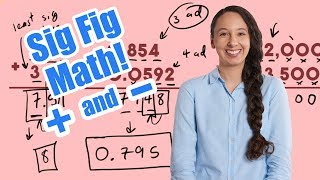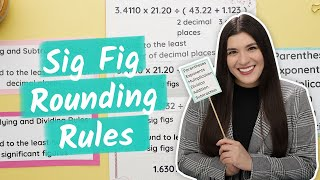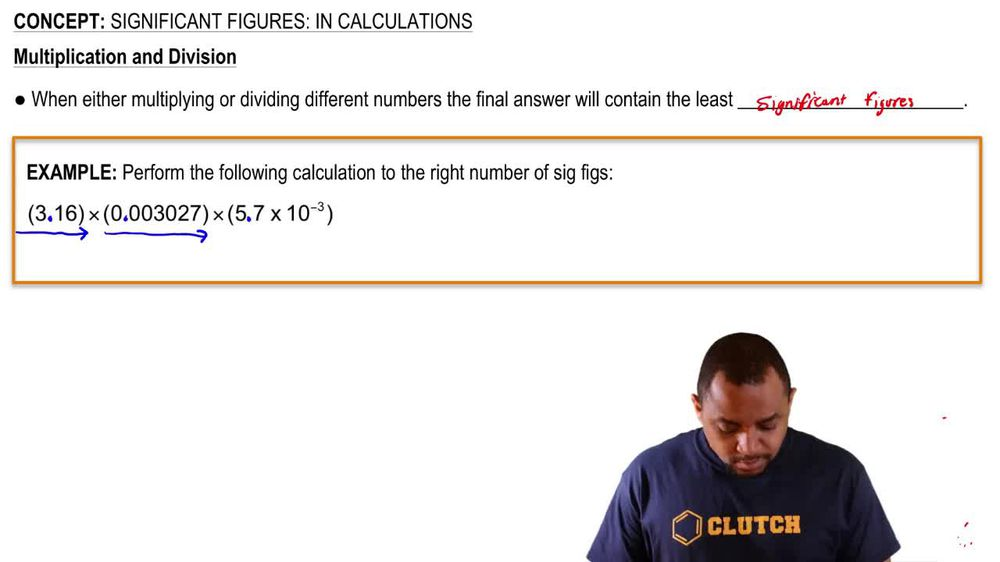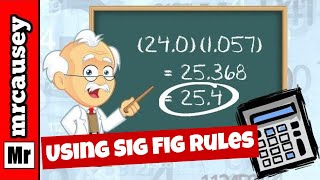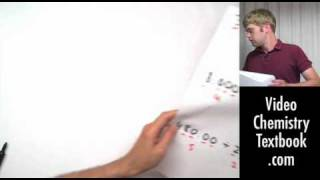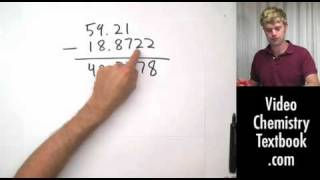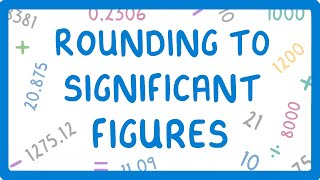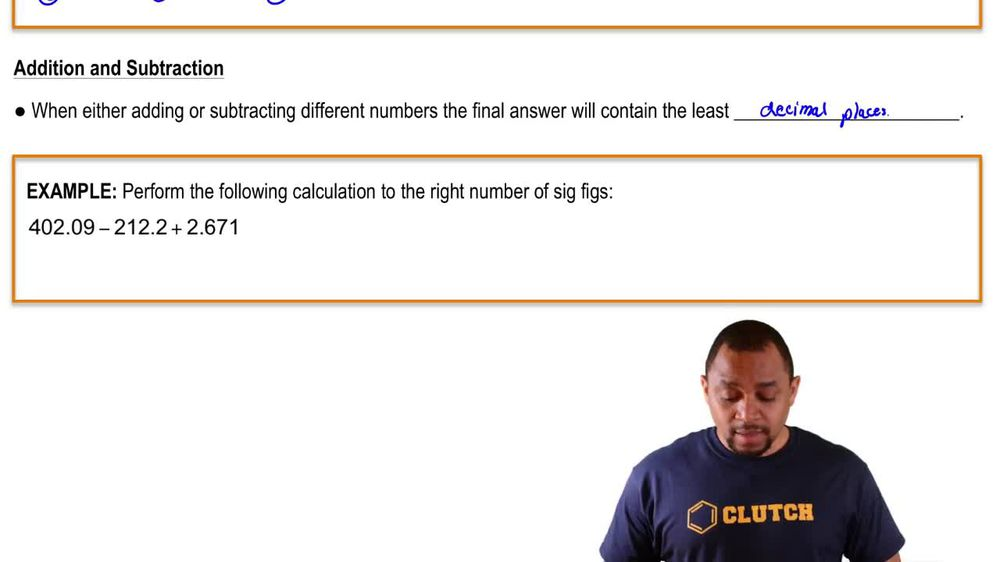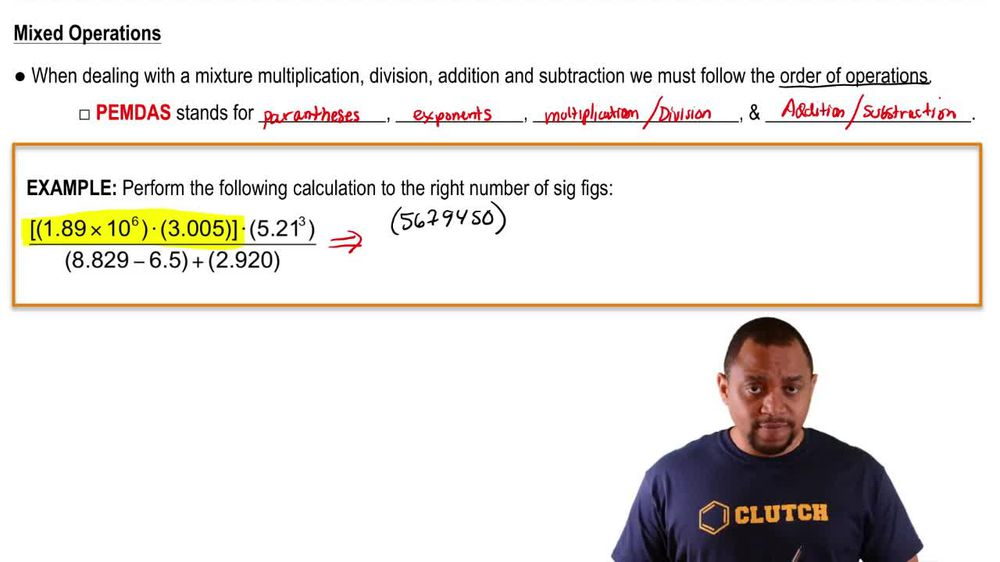Start typing, then use the up and down arrows to select an option from the list.
1. 1. Intro to General Chemistry2. Significant Figures: In Calculations
Problem

# Express the results of the following calculations with the correct number of significant figures. (e) 86.3 + 1.42 - 0.09

Relevant Solution1m
Play a video:
Hi everyone today. We have a question asking us to perform the following operation and we need to express the answer using the correct number of significant figures first let's just solve the operation. It equals .773. And now we need to remember our rules for significant figures for addition and subtraction and that is the least number of decimal places. So let's look at how many decimal places. Each number has 780 has zero, 6.97 has to and 81.197 has three. So where you're going to have zero decimal places? That means our 705.773. We need to take away this .773. So we need to look at our five here and to the right of it to determine if we need to round up or not. And because seven is above five we do need to round up So our answer here will be 706. Thank you for watching. Bye.